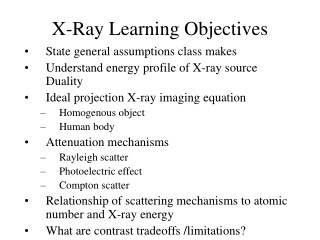DownloadDownload PresentationX-Ray Learning Objectives

# X-Ray Learning Objectives

Download Presentation## X-Ray Learning Objectives

- - - - - - - - - - - - - - - - - - - - - - - - - - - E N D - - - - - - - - - - - - - - - - - - - - - - - - - - -
##### Presentation Transcript

1. X-Ray Learning Objectives • State general assumptions class makes • Understand energy profile of X-ray source Duality • Ideal projection X-ray imaging equation • Homogenous object • Human body • Attenuation mechanisms • Rayleigh scatter • Photoelectric effect • Compton scatter • Relationship of scattering mechanisms to atomic number and X-ray energy • What are contrast tradeoffs /limitations?

2. X-Ray Learning Objectives • Describe the cause of source obliquity • How does incident energy vary due to source obliquity? How do we represent it mathematically? • How do we represent depth-dependent magnification mathematically? • How does geometric optics predict X-ray images will be distorted?

3. X-Ray Learning Objectives • What assumptions did we make to describe an X-ray system as a linear system? • Know and describe the linear system model of the X-ray in the space and frequency domain. • How does distortion change with object distance from the X-ray source? • How does depth dependent magnification change within the body?

4. X-Ray Learning Objectives • How does tilted source cause source magnification to vary over the image? • Describe constant velocity motion effects on imaging as linear system operator. • Define contrast and SNR with respect to detecting a lesion. • Describe SNR in terms of photon counts (e.g. SNR before the detector) and SNR after the X-ray detector.

5. X-Ray Detector Learning Objectives • Describe physically what causes the detector to degrade resolution. • Describe degradation as an impulse response akin to a low pass filter • Relate a cutoff frequency to the the detector • What engineering factors are involved in the cutoff frequency? • Define contrast and SNR with respect to detecting a lesion.

6. X-Ray SNR Learning Objectives • Describe SNR at various points along x-ray systems • After photons are captured by detector • After creating light photons • After creating film particles • After a photomultiplier tube • To what standards of gain does each section need to be designed?

7. X-Ray SNR Learning Objectives • Understand how reduced contrast from blurring of a lesion can reduce SNR ( Prob. 3 of Homework 3) • Be able to calculate SNR for problems like this.